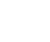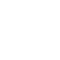# How to Calculate Dividend Yield

By: ,Dividend yield is the most commonly used measurement that income-seeking investors use to gauge the level of potential dividend income when evaluating investment options.

The formula to calculate the dividend yield is not very complex. It is a simple ratio of the total annual dividend distribution amount per share and the equity’s share price. This ratio is multiplied by 100 to get the dividend yield expressed as a percentage.

However, to calculate the dividend yield, investors must be careful to use the correct parameters and accurately interpret the results. While it is easy to calculate the dividend yield and gain basic information on the amount of earnings distributed to shareholders, dividend yield can be misleading and should be used in conjunction with other financial metrics and indicators when choosing investments.

### Dividend Yield

As a financial indicator, the dividend yield shows the share of the equity’s earnings – or assets in some cases – that the equity returns to its investors as periodic dividend distributions. While the yield could be calculated using total earnings and total market capitalization, it is generally calculated using the dividend payout per share and the share price. Using this method, we calculate the dividend yield by dividing the total annual dividend amount by the company’s share price.The resulting dividend yield is a ratio expressed as a decimal number. To express the dividend yield as percentage, we just multiply the entire expression by 100.While both calculations are simple, here is a specific example to illustrate the calculations using real dividend data.

Let’s look back to 2018. In 2018, AT&T, Inc. (NYSE:T) paid four quarterly dividends of \$0.50 each. The total annual dividend distribution for 2018 was \$2.00. At the end of trading on October 29, 2018, the share price closed at \$29.64. Using these figures, we can calculate the current dividend yield as:

Dividend Yield = \$2.00/\$29.64 = 0.0675 x 100 = 6.75%

### Trailing Dividend Yield

In addition to regular dividend distributions, equities occasionally distribute special dividends to dispense additional funds to their shareholders. These could be funds from dispositions of assets, legal settlements, divestitures, etc. Please note that these special dividends are not part of the dividend yield calculation. These special payments are not recurring and are not likely to be distributed the following year. Only regularly scheduled dividends factor into the formula to calculate the dividend yield.

The calculation for AT&T above used the total dividend payout from the previous year to calculate a trailing dividend yield. However, using the expected total dividend distribution for the upcoming 12-months, returns a forward dividend yield. To calculate the forward dividend yield, we first must calculate the annualized dividend payout based on the most recent quarterly distribution. There are few ways to do this calculation. If the dividend amount is the same for every period of the current year we can attempt to estimate the dividend hike for next year based on the equity’s specific dividend policy – if one exists – or extrapolate the potential increase based on the previous record.

### Forward Dividend Yield

While AT&T paid the same quarterly \$0.50 dividend throughout 2018, Texas Instruments, Inc. (NASDAQ:TXN) increased its dividend payout more than 24% for the last quarter.

Q1: \$0.62

Q2: \$0.62

Q3: \$0.62

Q4: \$0.77

Total 2018: \$2.63 per share

Texas Instruments paid a \$0.62 for the first three quarters and raised the quarterly payout to \$0.72 for the last period of 2018. Using the dividend amount for the fourth quarter, we can calculate the annualized dividend amount:

Annualized dividend = Most recent period’s dividend x No. of periods per year

Or, per the Texas Instruments example, the annualized dividend is:

Annualized dividend = \$0.72 x 4 = \$2.88

The \$2.88 total annualized dividend based on just the last period’s distribution is in this case 9.5% higher than the \$2.63 actual distribution for 2018.

Using the \$90.18 closing share price for Texas Instruments as of October 29, 2018, we can calculate the trailing and the forward dividend yields:

Forward Dividend Yield = \$2.88/\$90.18 = 0.0319 x 100 = 3.19%

Trailing Dividend Yield = \$2.63/\$90.18 = 0.0292 x 100 = 2.92%

The 3.19% forward yield is also 9.5% higher than the 2.92% trailing dividend yield.

### Using Dividend Yield for Selecting Investments

While it is relatively easy to calculate the dividend yield, the result could be very misleading about the performance of the potential equity. Because of its simplicity, the dividend yield does not provide any information about the underlying reasons for high or rising dividend yields. The yield can increase because the equity continues to boost its annual dividend distributions. Since the total annual dividend figure goes in the numerator of the dividend yield calculation, the dividend yield increase is directly proportional to the total annual dividend payout growth, which is a positive correlation for potential investors.

However, the dividend yield is inversely proportional to the equity’s share price – which goes in the formula’s denominator. Therefore, a dividend boost will be inversely proportional to the share-price increase. While it might be possible that the increased income from a higher yield could offset capital losses due to the declining share price, that scenario is very rare. The share prices fluctuate on a higher magnitude than dividend payouts. Therefore, the return from the rising dividend income is seldom enough to offset the asset depreciation.

To compensate for this shortcoming of the dividend yield, investors use additional indicators, such as the total return. The total return is a combined return from capital gains and dividend income, expressed as a percentage. This indicator normalizes return on investments or assets regardless of the source — asset appreciation or dividend income distributions.

An investment that had a 4% asset appreciation over the previous year and 4% trailing dividend yield over the same period would have a total return of 8% for the year. However, another equity that had a lower dividend yield of only 2%, but whose capital gain was 6%, has the same 8% total return for the year.

The definition of an acceptable level of dividend yield depends on multiple factors. While investors looking for pure income might insist on double-digit yields, investors looking for a balanced income and capital gain strategy might accept a dividend yield of 3% to 5%. Also, different sectors and industries have varied levels of average yields, which also should factor into the decision-making process.

While an important factor and a good starting point for analysis, investors must use additional financial indicators along with the yield to evaluate potential investments. Additionally, high dividend yields accompanied by high payout ratios could indicate an equity’s earnings distribution share that is too high, which could cause a shortage of cash flow necessary to support strategic investment or operational activities.

This article indicates why there is more to picking dividend income investments than just learning to calculate the dividend yield and selecting the equities with the highest yield. In addition to the yield, investors must consider the total return, the dividend payout ratio, as well as how different types of dividends are taxed to maximize overall returns, execute their investment strategies and reach their specific investment portfolio goals. However, learning how to calculate the dividend yield is a necessary first step.

Dividend increases and dividend decreases, new dividend announcements, dividend suspensions and other dividend changes occur daily. To make sure you don’t miss any important announcements, sign up for our. Let us do the hard work of gathering the data and sending the relevant information directly to your inbox.

In addition to E-mail Alerts, you will have access to our powerful dividend research tools. Take a quick video tour of the tools suite.

## Jonathan WolfgramConnect with Jonathan WolfgramJonathan Wolfgram is an investment analyst who writes website content at Eagle Financial Publications. He graduated from the University of Minnesota with Bachelor’s degrees in Finance and Philosophy. Jonathan writes for www.DividendInvestor.com and www.StockInvestor.com.
X
Search Dividend Investor